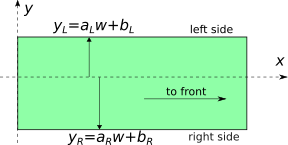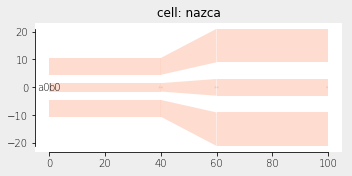Home Forums Nazca Connecting two dissimilar ribbons Reply To: Connecting two dissimilar ribbons

#5711

Dear mjbvrijn,

As of Nazca 0.5.4 a waveguide in a specific layer inside a xsection can be defined by providing the left edge and right edge of the waveguide independently. Multiple of such guides can be defined inside a single xsection. The edge is define by y = a*w+b, as clarified in the picture below. Parameter w is the width setting of the xsection.As long as you can describe an edge of by a single y = a*w +b statement, a standard inteconnect taper will work for a case as you describe, as shown in the next example. Note that a and b parameters for an edge are provided as tuples (a, b) via the keywords leftedge and rightedge:

``````import nazca as nd

nd.add_layer2xsection(xsection='GSG', leftedge=(0.5, 0), rightedge=(-0.5, 0), layer='L1')
nd.add_layer2xsection(xsection='GSG', leftedge=(3.5, 0), rightedge=(1.5, 0), layer='L1')

tr.strt(length=40).put(0)
tr.taper(length=20, width2=6.0).put()
tr.strt(length=40, width=6.0).put()

nd.export_plt()``````If you start with the dimension on the left (y1) and right (y2) side of the taper in the above example you can find a and b of an edge as follows:

y1 = a1 * w1 + b1

y2 = a2 * w2 + b2

and solve for y1 =  y2

This gives

a = (y1 – y2) / (w1 – w2)

b = (y2*w1 – y1*w2) / (w1 – w2)

and note that the widths w1 and w2 can be considered scaling numbers instead of actual widths of a guide in the xsection.

Ronald